NCERT Solutions for Class 8 Maths Chapter 1 Rational Numbers Ex 1.1 are part of NCERT Solutions for Class 8 Maths. Here we have given NCERT Solutions for Class 8 Maths Chapter 1 Rational Numbers Ex 1.1.

 Board CBSE Textbook NCERT Class Class 8 Subject Maths Chapter Chapter 1 Chapter Name Rational Numbers Exercise Ex 1.1 Number of Questions Solved 11 Category NCERT Solutions

## NCERT Solutions for Class 8 Maths Chapter 1 Rational Numbers Ex 1.1

Question 1.
Using appropriate properties find:
(i) $$-\frac { 2 }{ 5 } \times \frac { 3 }{ 5 } +\frac { 5 }{ 2 } -\frac { 3 }{ 5 } \times \frac { 1 }{ 6 }$$
(ii) $$\frac { 2 }{ 5 } \times \left( -\frac { 3 }{ 7 } \right) -\frac { 1 }{ 6 } \times \frac { 3 }{ 2 } +\frac { 1 }{ 14 } \times \frac { 2 }{ 5 }$$
Solution.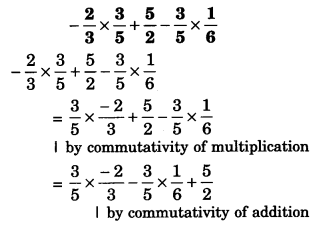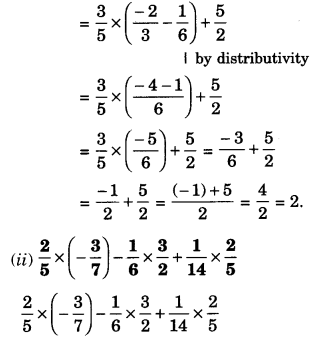Question 2.
Write the additive inverse of each of the following:Solution.
(i) $$\frac { 2 }{ 8 }$$
Additive inverse of $$\frac { 2 }{ 8 }$$ is $$\frac { 2 }{ 8 }$$

(ii) $$-\frac { 5 }{ 9 }$$
$$\frac { -6 }{ -5 } =\frac { 6 }{ 5 }$$
Additive inverse of $$\frac { -6 }{ -5 }$$ is $$\frac { -6 }{ 5 }$$

(iii) $$\frac { -6 }{ -5 }$$
$$\frac { -6 }{ -5 }$$=$$\frac { 6 }{ 5 }$$
Additive inverse of $$\frac { -6 }{ -5 }$$ is $$\frac { -6 }{ 5 }$$

(iv) $$\frac { 2 }{ -9 }$$
Additive inverse of $$\frac { 2 }{ -9 }$$ is $$\frac { 2 }{ 9 }$$

(v) $$\frac { 19 }{ -6 }$$
Additive inverse of  $$\frac { 19 }{ -6 }$$ is $$\frac { 19 }{ 6 }$$

Question 3.
Verify that – (-x) = x for :
(i) $$x=\frac { 11 }{ 15 }$$
(ii) $$x=-\frac { 13 }{ 17 }$$
Solution.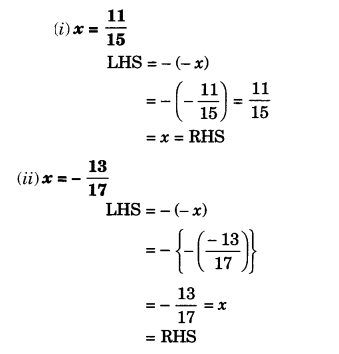Question 4.
Find the multiplicative inverse of the following: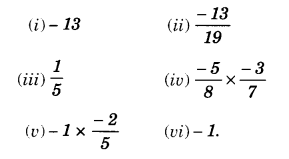Solution.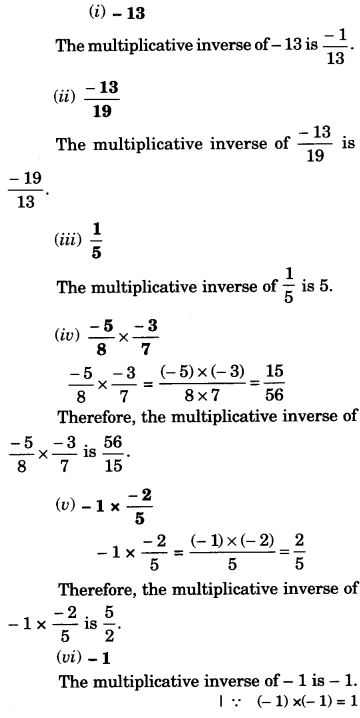Question 5.
Name the property under multiplication used in each of the following:
(i) $$\frac { -4 }{ 5 } \times \left( 1 \right) =1\times \frac { -4 }{ 5 } =-\frac { 4 }{ 5 }$$
(ii) $$-\frac { 13 }{ 17 } \times \frac { -2 }{ 7 } =\frac { -2 }{ 7 } \times \frac { -13 }{ 17 }$$
(iii) $$\frac { -19 }{ 29 } \times \frac { 29 }{ -19 } =1$$
Solution.
(i) 1 is the multiplicative identity
(ii) Commutativity of multiplication
(iii) Multiplicative inverse.

Question 6.
Multiply $$\frac { 6 }{ 13 }$$ by the reciprocal of $$\frac { -7 }{ 16 }$$
Solution.
Reciprocal of $$\frac { -7 }{ 16 }$$ is $$\frac { -16 }{ 7 }$$
Now,
$$\frac { 6 }{ 13 } \times \frac { -16 }{ 7 } =\frac { 6\times \left( -16 \right) }{ 13\times 7 } =\frac { -96 }{ 91 }$$

Question 7.
Tell what property allows you to compute : $$\frac { 1 }{ 3 } \times \left( 6\times \frac { 4 }{ 3 } \right)$$ as $$\left( \frac { 1 }{ 3 } \times 6 \right) \times \frac { 4 }{ 3 }$$
Solution.
Associativity.

Question 8.
Is the $$\frac { 8 }{ 9 }$$ multiplicative inverse of $$-1\frac { 1 }{ 8 }$$ ? Why or why not?
Solution.
$$-1\frac { 1 }{ 8 } =-\frac { 9 }{ 8 }$$
Now, $$\frac { 8 }{ 9 } \times \frac { -9 }{ 8 } =-1\neq 1$$
So, No ; $$\frac { 8 }{ 9 }$$ is not the multiplicative inverse of $$-1\frac { 1 }{ 8 } \left( =-\frac { 9 }{ 8 } \right)$$ because the product of $$\frac { 8 }{ 9 }$$ and -13(-) and $$-1\frac { 1 }{ 8 } \left( =-\frac { 9 }{ 8 } \right)$$ is not 1.

Question 9.
Is 0.3 the multiplicative inverse of $$3\frac { 1 }{ 3 }$$ ? Why or why not?
Solution.
Yes ; 0.3 is the multiplicative inverse of $$\frac { 10 }{ 3 }$$ because
$$\frac { 3 }{ 10 } \times \frac { 10 }{ 3 } =\frac { 3\times 10 }{ 10\times 3 } =\frac { 30 }{ 30 } =1$$

Question 10.
Write :
(i) The rational number that does not have a reciprocal.
(ii) The rational numbers that are equal to their reciprocals.
(iii) The rational number that is equal to its negative.
Solution.
(i) The rational number ‘0′ does not have a reciprocal.
(ii) The rational numbers 1 and (-1) are equal to their own reciprocals.
(iii) The rational number 0 is equal to its negative.

Question 11.
Fill in the blanks :
(i) Zero has……….reciprocal.
(ii) The numbers……….and………are their own reciprocals.
(iii) The reciprocal of – 5 is.………….
(iv) Reciprocal of $$\frac { 1 }{ x }$$, where $$x\neq 0$$
(v) The product of two rational numbers is always a.………
(vi) The reciprocal of a positive rational number is……….
Solution.
(i) Zero has no reciprocal.
(ii) The numbers 1 and -1 are their own reciprocals.
(iii) The reciprocal of – 5 is $$-\frac { 1 }{ 5 }$$
(iv) Reciprocal of $$\frac { 1 }{ x }$$, where $$x\neq 0$$ is x.
(v) The product of two rational numbers is always a rational number.
(vi) The reciprocal of a positive rational number is positive.

We hope the NCERT Solutions for Class 8 Maths Chapter 1 Rational Numbers Ex 1.1 help you. If you have any query regarding NCERT Solutions for Class 8 Maths Chapter 1 Rational Numbers Ex 1.1, drop a comment below and we will get back to you at the earliest.